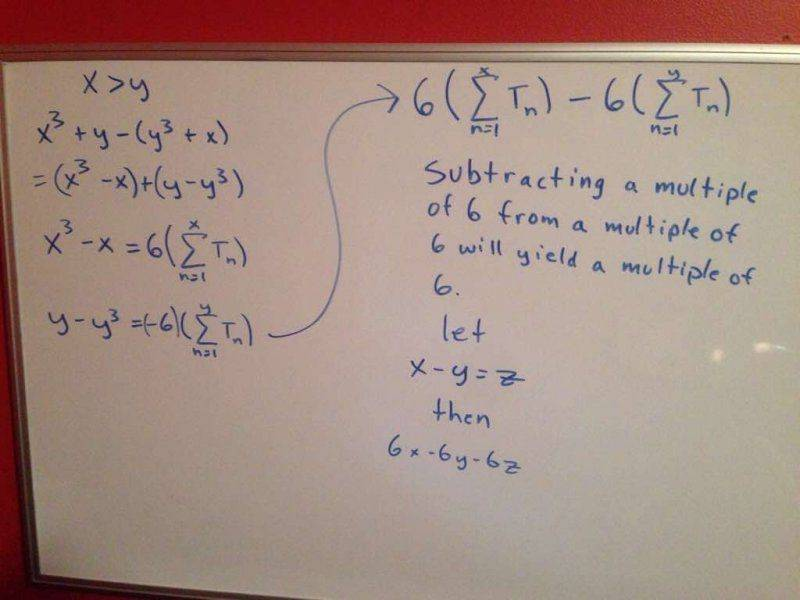# Prove that the difference of x^3+y and y^3+x is a always a multiple of 6

• willr12

#### willr12

I recently came across a problem that said 'prove that the difference of x^3+y and y^3+x is a always a multiple of 6, given that x and y are integers'. I tried it, but I'm not sure if this is a valid proof.this is with the first triangular number being 0 and Tsubn being the nth triangular number.

If you can use or prove the formula for the triangular numbers, sure.

Or you can do this:
x3 + y - (y3 + x) = x3 - x - (y3 - y)
= x(x2 - 1) - y(y2 - 1)
= x(x - 1)(x + 1) - y(y - 1)(y+ 1)

In a more suggestive form, the last expression is
(x - 1)(x)(x + 1) - (y - 1)(y)(y + 1), with each group being the product of three successive integers.

It's not too hard to show that the product of three integers in succession is divisible by 6 (can do this by induction), so the difference of two such products is also divisible by 6.

(can do this by induction)
Or splitting it into 5 to 6 cases.
That is the more elementary approach if the triangle formula is not available.

No need to consider different cases . Given 3 consecutive integers, exactly one is a multiple of 3 and at least one is even. Therefore the product is divisible by both 2 and 3.